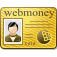Replenishment date: 18.02.2023
Content: Econometrics_MMA_test with answers.docx (235.85 KB)
️Automatic issue of goods ✔️
Sales:
4
Refunds:
0
Reviews:
0
Views:
84SellerSeller:
alevtina_sar
Rating:
2,12
Report a violation
Description
Find the mathematical expectation of a discrete random variable X given by the following distribution law

a.102

b.1

c.60

d.204

The distribution of a discrete random variable X is given. Fill in the missing element in the table

If the random variables X and Y are independent, then the coefficient of covariance is:

a.-1

b.1

c.0

d. does not exist

The linear correlation coefficient of the two quantities is 0,94.

Which statement is true?

a. there is a weak direct linear relationship between variables

b.weak linear feedback between variables

c. there is a strong direct linear relationship between variables

d. there is no linear relationship between variables

What is the correlation coefficient if cov(x,y)=10, Var(x)=25, Var(y)=16

a.0,2

b.0,3

c.0,1

d.0,5

The mathematical expectation of the squared deviation of a random variable from its mathematical expectation is:

a. standard deviation of a random variable

b. random variable variance

c. random variable correlation

d.covariance of a random variable

The maximum value of the correlation coefficient:

a.0,5

b.plus infinity

c.0

d.1

Specify the correct property of the sample covariance coefficient

a.cov(x,y) = Var2(x)

b.cov(x,x) = Var(x)

c.cov(x,x) = Var2(x)

d.cov(a,x)= a, a=const

The area under the graph of the probability density function of the normal distribution is

a.0

b.0,025

c.1

d.0,5

To check the autocorrelation of residuals, apply:

a. series test based on sample median

b. criterion of ascending and descending series

c.irwin method

d. Durbin-Watson statistics

The estimated value of the Durbin-Watson statistics is 1,5. Critical values ​​(5%): dL = 1,2, dU= 1,4. Observed:

a.positive autocorrelation

b.negative autocorrelation

c. zone of uncertainty

d.no autocorrelation

The significance of the trend model coefficients is checked using:

a.asymmetry coefficient

b. Durbin-Watson statistics

c. kurtosis coefficient

Student's dt-test

The maximum value of the Durbin-Watson statistics:

a.4

b.2

c.3

d.1

The estimated value of the Durbin-Watson statistics is 2,57. Critical values ​​(5%): dL = 1,1, dU= 1,34. Observed:

a.no autocorrelation

b.positive autocorrelation

c.negative autocorrelation

d. zone of uncertainty

The estimated value of the Durbin-Watson statistics is 2,87. Critical values ​​(5%): dL = 1,1, dU= 1,34. Observed:

a.negative autocorrelation

b.no autocorrelation

c. zone of uncertainty

d.positive autocorrelation

If there is no autocorrelation of the residuals, then the Durbin-Watson statistic is close to:

a.4

b.1

c.0

d.2

If there is a negative autocorrelation of the residuals, then the Durbin-Watson statistic is close to:

a.2

b.4

c.1

d.3

The skewness coefficient in the study of residues is used to check:

a. correlation of residuals

b. trend in balances

c.normal distribution of a number of residues

d. relative error of a series of residuals

The correlation coefficient between variables x and y is 0,68. What is the coefficient of determination of the paired linear regression y on x (with a constant).

a.0,4624

b.0,6424

c.0,32

d.0,72
Find the mathematical expectation of a discrete random variable X given by the following distribution law

a.102

b.1

c.60

d.204

The distribution of a discrete random variable X is given. Fill in the missing element in the table

If the random variables X and Y are independent, then the coefficient of covariance is:

a.-1

b.1

c.0

d. does not exist

The linear correlation coefficient of the two quantities is 0,94.

Which statement is true?

a. there is a weak direct linear relationship between variables

b.weak linear feedback between variables

c. there is a strong direct linear relationship between variables

d. there is no linear relationship between variables

What is the correlation coefficient if cov(x,y)=10, Var(x)=25, Var(y)=16

a.0,2

b.0,3

c.0,1

d.0,5

The mathematical expectation of the squared deviation of a random variable from its mathematical expectation is:

a. standard deviation of a random variable

b. random variable variance

c. random variable correlation

d.covariance of a random variable

The maximum value of the correlation coefficient:

a.0,5

b.plus infinity

c.0

d.1

Specify the correct property of the sample covariance coefficient

a.cov(x,y) = Var2(x)

b.cov(x,x) = Var(x)

c.cov(x,x) = Var2(x)

d.cov(a,x)= a, a=const

The area under the graph of the probability density function of the normal distribution is

a.0

b.0,025

c.1

d.0,5

To check the autocorrelation of residuals, apply:

a. series test based on sample median

b. criterion of ascending and descending series

c.irwin method

d. Durbin-Watson statistics

The estimated value of the Durbin-Watson statistics is 1,5. Critical values ​​(5%): dL = 1,2, dU= 1,4. Observed:

a.positive autocorrelation

b.negative autocorrelation

c. zone of uncertainty

d.no autocorrelation

The significance of the trend model coefficients is checked using:

a.asymmetry coefficient

b. Durbin-Watson statistics

c. kurtosis coefficient

Student's dt-test

The maximum value of the Durbin-Watson statistics:

a.4

b.2

c.3

d.1

The estimated value of the Durbin-Watson statistics is 2,57. Critical values ​​(5%): dL = 1,1, dU= 1,34. Observed:

a.no autocorrelation

b.positive autocorrelation

c.negative autocorrelation

d. zone of uncertainty

The estimated value of the Durbin-Watson statistics is 2,87. Critical values ​​(5%): dL = 1,1, dU= 1,34. Observed:

a.negative autocorrelation

b.no autocorrelation

c. zone of uncertainty

d.positive autocorrelation

If there is no autocorrelation of the residuals, then the Durbin-Watson statistic is close to:

a.4

b.1

c.0

d.2

If there is a negative autocorrelation of the residuals, then the Durbin-Watson statistic is close to:

a.2

b.4

c.1

d.3

The skewness coefficient in the study of residues is used to check:

a. correlation of residuals

b. trend in balances

c.normal distribution of a number of residues

d. relative error of a series of residuals

The correlation coefficient between variables x and y is 0,68. What is the coefficient of determination of the paired linear regression y on x (with a constant).

a.0,4624

b.0,6424

c.0,32

d.0,72
Recommended